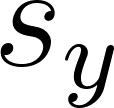×
Get Full Access to Probability And Statistical Inference - 9 Edition - Chapter 7.2 - Problem 3e
Get Full Access to Probability And Statistical Inference - 9 Edition - Chapter 7.2 - Problem 3e

×

# Independent random samples of the heights of adult malesISBN: 9780321923271 41

## Solution for problem 3E Chapter 7.2

Probability and Statistical Inference | 9th Edition

• Textbook Solutions
• 2901 Step-by-step solutions solved by professors and subject experts
• Get 24/7 help from StudySoup virtual teaching assistantsProbability and Statistical Inference | 9th Edition

4 5 1 402 Reviews
29
4
Problem 3E

Independent random samples of the heights of adult males living in two countries yielded the following results: $$n=12, \bar{x}=65.7$$ inches, $$s_{x}=4$$ inches and $$m=15, \bar{y}=68.2$$ inches, $$s_{y}=3$$ inches. Find an approximateconfidence interval for the difference $$\mu_{X}-\mu_{Y}$$ of the means of the populations of heights. Assume that $$\sigma_{X}^{2}=\sigma_{Y}^{2}$$

Equation Transcription:Text Transcription:

n=12, bar x=65.7

s_x=4

m=15,bar y=68.2

s_y=3

mu_X-mu_Y

sigma_X^2=sigma_Y^2

Step-by-Step Solution:

Step 1 of 2

Independent random samples of the heights of adult males living in two countries yielded the following results: n=12,=65.7 inches, sx=4 inches and m =15,=68.2 inches,=3 inches. Find an approximate 98% confidence interval for the differenceof the means of the populations of heights. Assume that.

Step 2 of 2

##### ISBN: 9780321923271

This full solution covers the following key subjects: inches, heights, interval, confidence, countries. This expansive textbook survival guide covers 59 chapters, and 1476 solutions. The answer to “?Independent random samples of the heights of adult males living in two countries yielded the following results: $$n=12, \bar{x}=65.7$$ inches, $$s_{x}=4$$ inches and $$m=15, \bar{y}=68.2$$ inches, $$s_{y}=3$$ inches. Find an approximate confidence interval for the difference $$\mu_{X}-\mu_{Y}$$ of the means of the populations of heights. Assume that $$\sigma_{X}^{2}=\sigma_{Y}^{2}$$Equation Transcription: Text Transcription:n=12, bar x=65.7s_x=4m=15,bar y=68.2 s_y=3mu_X-mu_Y sigma_X^2=sigma_Y^2” is broken down into a number of easy to follow steps, and 56 words. Since the solution to 3E from 7.2 chapter was answered, more than 408 students have viewed the full step-by-step answer. The full step-by-step solution to problem: 3E from chapter: 7.2 was answered by , our top Statistics solution expert on 07/05/17, 04:50AM. This textbook survival guide was created for the textbook: Probability and Statistical Inference , edition: 9. Probability and Statistical Inference was written by and is associated to the ISBN: 9780321923271.

Unlock Textbook Solution